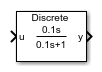# Washout (Discrete or Continuous)

Discrete-time or continuous-time washout or high-pass filter

• Library:
• Simscape / Electrical / Control / General Control

•## Description

The Washout (Discrete or Continuous) block implements a washout filter in conformance with IEEE 421.5-2016. The washout is also known as a high-pass filter.

You can switch between continuous and discrete implementations of the integrator using the Sample time parameter.

### Equations

Continuous

To configure the Washout (Discrete or Continuous) block for continuous time, set the Sample time property to `0`. This representation is equivalent to the continuous transfer function:

`$G\left(s\right)=\frac{Ts}{Ts+1},$`

where T is the time constant. From the preceding transfer function, the washout defining equations are:

`$\left\{\begin{array}{c}\stackrel{˙}{x}\left(t\right)=\frac{1}{T}\left(-x\left(t\right)+u\left(t\right)\right)\\ y\left(t\right)=-x\left(t\right)+u\left(t\right)\end{array}\text{\hspace{0.17em}}\text{\hspace{0.17em}}x\left(0\right)={u}_{0},\text{\hspace{0.17em}}y\left(0\right)=0,$`

where:

• u is the washout input.

• x is the washout state.

• y is the washout output.

• t is the simulation time.

• u0 is the initial input to the block.

Discrete

To configure the washout Washout (Discrete or Continuous) for discrete time, set the Sample time property to a positive, nonzero value, or to `-1` to inherit the sample time from an upstream block. The discrete representation is equivalent to the transfer function:

`$G\left(z\right)=\frac{z-1}{z+{T}_{s}/T-1},$`

where Ts is the sample time. From the discrete transfer function, the washout defining equations are defined using the forward Euler method:

`$\left\{\begin{array}{c}x\left(n+1\right)=\left(1-\frac{{T}_{s}}{T}\right)x\left(n\right)+\left(\frac{{T}_{s}}{T}\right)u\left(n\right)\\ y\left(n\right)=u\left(n\right)-x\left(n\right)\end{array}\text{\hspace{0.17em}}\text{\hspace{0.17em}}x\left(0\right)={u}_{0},\text{\hspace{0.17em}}y\left(0\right)=0,$`

where:

• u is the washout input.

• x is the washout state.

• y is the washout output.

• n is the simulation time step.

• u0 is the initial input to the block.

### Initial Conditions

To specify the initial conditions of this block, set Initialization to:

• `Inherited from block input` — The block sets the state and output initial conditions to the initial input.

• `Specify as parameter` — The block sets the state initial condition to the value of Initial state.

### Bypass Filter Dynamics

Set the time constant to a value smaller than or equal to the sample time to ignore the dynamics of the filter. When bypassed, the block feeds the input directly to the output:

`$T\le {T}_{s}\to y=u\text{\hspace{0.17em}}.$`

In the continuous case, the sample time and time constant must both be zero.

## Ports

### Input

expand all

Washout input signal. The block uses the input initial value to determine the state initial value.

Data Types: `single` | `double`

### Output

expand all

Washout output signal.

Data Types: `single` | `double`

## Parameters

expand all

Washout time constant. Set this value less than the Sample time to bypass the dynamics of the filter.

Specify the initial state condition for this block. For more information, see Initial Conditions.

Block initial state.

#### Dependencies

To enable this parameter, set Initialization to `Specify as parameter`.

Time between consecutive block executions. During execution, the block produces outputs and, if appropriate, updates its internal state. For more information, see What Is Sample Time? and Specify Sample Time.

For inherited discrete-time operation, specify `-1`. For discrete-time operation, specify a positive integer. For continuous-time operation, specify `0`.

If this block is in a masked subsystem, or other variant subsystem that allows you to switch between continuous operation and discrete operation, promote the sample time parameter. Promoting the sample time parameter ensures correct switching between the continuous and discrete implementations of the block. For more information, see Promote Block Parameters on a Mask.

 IEEE Recommended Practice for Excitation System Models for Power System Stability Studies. IEEE Std 421.5-2016. Piscataway, NJ: IEEE-SA, 2016.